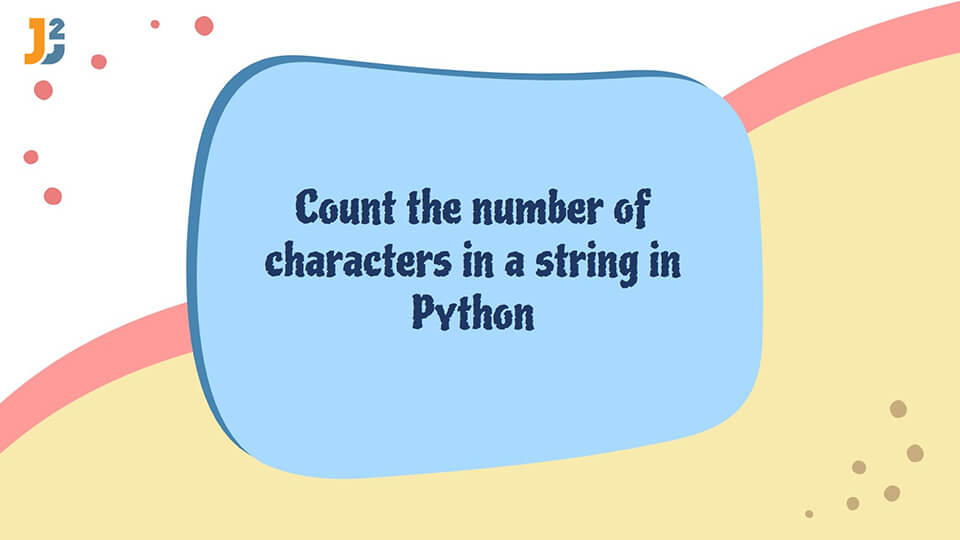# Count number of characters in a string in pythonIn this post, we will see how to count number of characters in a String in Python.

We can think of strings as a collection of characters, with every character at a given index.

## Ways to count the number of characters in a string in Python

In this tutorial, we will find out the total number of characters in a string in Python.

### Using the `len()` function

This function is the most straightforward method. The `len()` function returns the length of a given iterable in Python. We can use it to find the number of characters in a string.

For example,

Output:

9

### Using the `for` loop

We can use the `for` loop to iterate over a string in Python. We can use a counter variable and increment it in every iteration. This variable will return the total number of characters in a string.

For example,

Output:

9

In the above example,

• The `t` variable is given a value of 0.
• We iterate over the string `s` using the `for` loop.
• In every iteration, we increment `t` and display its value after the loop ends.

### Using the `collections.Counter` class

The `collections.Counter` class stores the elements of a string as key-value pairs. The keys are the characters of the string, and the value of each key is how many times this character occurs in the string.

We can sum up these values to find the total number of characters in the given string.

See the code below.

Output:

9

In the above code,

• We create an object of the `Counter` class `ob`.
• We create an object of all the values of the dictionary-like object `ob` with the `values()` function.
• The `sum()` function returns the sum of these values.

## Conclusion

In this tutorial, we discussed how to get the characters in a given string in Python. The `len()` function is the simplest and most used method. We can also use the `for` loop and `Counter` class for a lengthy method.

import_contacts

### You may also like:## Related Posts

•28 October

### How to decrement for loop in python

Table of ContentsWhat is for loop in Python?Ways to decrement the for loop in PythonUsing the start, stop and step parameters in range() functionUsing the reversed() functionUsing the while loopConclusion We use the for loop widely in the programming world. Most programs, whether highly complex or not, contain this loop. Sometimes, depending on the conditions, […]

•19 June

### Calculator program in Python

Table of ContentsUsing the while loop along with the if...else conditional statement.Define functions for Addition, Subtraction, Multiplication and DivisionTake user input using input functionComplete calculator program in Python A simple Calculator can be utilized to carry out the four basic arithmetic operations namely addition, division, multiplication, and subtraction depending on the input of the user. […]

•07 June

### Number guessing game in Python

Table of ContentsNumber guessing game RulesNumber guessing game implementation in PythonJava implementation A number guessing game is a common mini-project for basic programmers who have a grasp on random number generation and conditional statements with iteration. The number guessing game is based on a concept where player has to guess a number between given range. […]

•31 May

### Perfect number program in Python

Table of ContentsUse the Simple iteration method to check whether a given number is a perfect number.Use the square root method to check whether a given number is a perfect number. According to number theory, a limb of pure mathematics that deals with integers, a Perfect Number can be defined as a positive integer whose […]

## Subscribe to our newletter

Get quality tutorials to your inbox. Subscribe now.